# 数据结构与算法（java）

### 简述

`数据结构+算法＝程序`

• 如何有效地存储数据，不同的数据结构产生什么样的算法复杂性，有没有更好的存储方法提高算法的效率？

### 基本的概念

`数据结构的三种层次`

1. 逻辑结构--抽象层： 主要描述的是数据元素之间的逻辑关系
• `集合结构（集）` 所有的元素都属于一个总体，除了同属于一个集合外没有其他关系。集合结构不强调元素之间的任何关联性。
• `线性结构（表）` 数据元素之间具有一对一的前后关系。结构中必须存在唯一的首元素和唯一的尾元素。
• `树形结构（树）` 数据元素之间一对多的关系
• `网状结构（图）` 图状结构或网状结构 结构中的数据元素之间存在多对多的关系

1. 物理结构--存储层： 主要描述的是数据元素之间的位置关系
• `顺序结构` 顺序结构就是使用一组连续的存储单元依次存储逻辑上相邻的各个元素
`优点` 只需要申请存放数据本身的内存空间即可，支持下标访问，也可以实现随机访问。
`缺点` 必须静态分配连续空间，内存空间的利用率比较低。插入或删除可能需要移动大量元素，效率比较低

• `链式结构` 链式存储结构不使用连续的存储空间存放结构的元素，而是为每一个元素构造一个节点。节点中除了存放数据本身以外，还需要存放指向下一个节点的指针。
`优点` 不采用连续的存储空间导致内存空间利用率比较高，克服顺序存储结构中预知元素个数的缺点 插入或删除元素时，不需要移动大量的元素。
`缺点` 需要额外的空间来表达数据之间的逻辑关系， 不支持下标访问和随机访问。

• `索引结构` 除建立存储节点信息外，还建立附加的索引表来标节点的地址。索引表由若干索引项组成。
`优点` 是用节点的索引号来确定结点存储地址，检索速度块
`缺点： 增加了附加的索引表,会占用较多的存储空间。

• `散列结构` 由节点的关键码值决定节点的存储地址。散列技术除了可以用于查找外，还可以用于存储。
`优点` 散列是数组存储方式的一种发展，采用存储数组中内容的部分元素作为映射函数的输入，映射函数的输出就是存储数据的位置, 相比数组，散列的数据访问速度要高于数组
`缺点` 不支持排序，一般比用线性表存储需要更多的空间，并且记录的关键字不能重复。

1. 运算结构--实现层： 主要描述的是如何实现数据结构
• 分配资源，建立结构，释放资源
• 插入和删除
• 获取和遍历
• 修改和排序

O符号

O在算法当中表述的是时间复杂度，它在分析算法复杂性的方面非常有用。常见的有：

1. `O(1)`：最低的复杂度，无论数据量大小，耗时都不变，都可以在一次计算后获得。哈希算法就是典型的O(1)
2. `O(n)`：线性，n表示数据的量，当量增大，耗时也增大，常见有遍历算法
3. `O(n²)`：平方，表示耗时是n的平方倍，当看到循环嵌循环的时候，基本上这个算法就是平方级的，如：冒泡排序等
4. `O(log n)`：对数，通常ax=n,那么数x叫做以a为底n的对数,也就是x=logan，这里是a通常是2，如：数量增大8倍，耗时只增加了3倍，二分查找就是对数级的算法，每次剔除一半
5. `O(n log n)`：线性对数，就是n乘以log n,按照上面说的数据增大8倍，耗时就是8*3=24倍，归并排序就是线性对数级的算法

### Array

``````//只声明了类型和长度

//声明了类型，初始化赋值，大小由元素个数决定

``````

`大小固定，不能动态扩展(初始化给大了，浪费；给小了，不够用)，插入快，删除和查找慢`

``````public class Array {
private int[] intArray;

private int elems;

private int length;

public Array(int max) {
length = max;
intArray = new int[max];
elems = 0;
}

/**
* 添加
* @param value
*/
if(elems == length){
System.out.println("error");
return;
}
intArray[elems] = value;
elems++;
}

/**
* 查找
* @param searchKey
* @return
*/
public int find(int searchKey){
int i;
for(i = 0; i < elems; i++){
if(intArray[i] == searchKey)
break;
}
if(i == elems){
return -1;
}
return i;
}

/**
* 删除
* @param value
* @return
*/
public boolean delete(int value){
int i = find(value);
if(i == -1){
return false;
}
for (int j = i; j < elems-1; j++) {
//后面的数据往前移
intArray[j] = intArray[j + 1];
}
elems--;
return true;
}

/**
* 更新
* @param oldValue
* @param newValue
* @return
*/
public boolean update(int oldValue,int newValue){
int i = find(oldValue);
if(i == -1){
return false;
}
intArray[i] = newValue;
return true;
}

/**
* 显示所有
*/
public void display(){
for(int i = 0 ; i < elems ; i++){
System.out.print(intArray[i]+" ");
}
System.out.print("\n");
}

/**
* 冒泡排序
* 每趟冒出一个最大数/最小数
* 每次运行数量：总数量-运行的趟数(已冒出)
*/
public void bubbleSort(){
for(int i = 0; i < elems-1; i++){//排序趟数  n-1次就行了
System.out.println("第"+(i+1)+"趟：");
for(int j = 0; j < elems-i-1; j++){//每趟次数 (n-i) -1是防止下标越界，后面赋值用到了+1
if(intArray[j] > intArray[j+1]){ //控制按大冒泡还是按小冒泡
int temp = intArray[j];
intArray[j] =  intArray[j+1];
intArray[j+1] = temp;
}
display();
}
}
}

/***
* 选择排序
* 每趟选择一个最大数/最小数
* 每次运行数量：总数量-运行的趟数(已选出)
*/
public void selectSort(){
for(int i = 0; i < elems-1; i++) {//排序趟数  n-1次就行了
int min = i;
for(int j = i+1; j < elems; j++){ //排序次数 每趟选择一个数出来，-1次
if(intArray[j] < intArray[min]){
min = j;
}
}
if(i != min ){
int temp = intArray[i];
intArray[i] = intArray[min];
intArray[min] = temp;
}
display();
}
}

/**
* 插入排序
* 每趟选择一个待插入的数
* 每次运行把待插入的数放在比它大/小后面
*/
public void insertSort(){
int j;
for(int i = 1; i < elems; i++){
int temp = intArray[i];
j = i;
while (j > 0 && temp < intArray[j-1]){
intArray[j] = intArray[j-1];
j--;
}
intArray[j] = temp;
}
display();
}

public static void main(String[] args) {
Array array = new Array(10);
//        array.bubbleSort();
//        array.selectSort();
array.insertSort();
//        array.display();
//        System.out.println(array.find(4));
//        System.out.println(array.delete(1));
//        array.display();
//        System.out.println(array.update(2,6));
//        array.display();
}
}
``````

### Stack

• 栈（stack）又称为堆栈或堆叠，栈作为一种数据结构，它按照先进后出的原则存储数据，先进入的数据被压入栈底，最后的数据在栈顶
• java中Stack是Vector的一个子类，只定义了默认构造函数，用来创建一个空栈。
• 栈是元素的集合，其包含了两个基本操作：push 操作可以用于将元素压入栈，pop 操作可以将栈顶元素移除。
• 遵循后入先出（LIFO）原则。
• 时间复杂度:
• 索引: `O(n)`
• 搜索: `O(n)`
• 插入: `O(1)`
• 移除: `O(1)`
``````Stack()
``````

``````public class Stack {
//小贴士：通常可以利用栈实现字符串逆序，还可以利用栈判断分隔符是否匹配，如<a[b{c}]>，可以正进反出，栈为空则成功。

/**基于数组实现的顺序栈，连续存储的线性实现，需要初始化容量**/
//固定数据类型
//private int[] array;
//动态数据类型
private Object[] objArray;

private int maxSize;

private int top;

public Stack() {
}

public Stack(int maxSize) {
if(maxSize > 0){
objArray = new Object[maxSize];
this.maxSize = maxSize;
top = -1;
}else{
throw new RuntimeException("初始化大小错误：maxSize=" + maxSize);
}
}

/**
* 入栈
* @param obj
*/
public void objPush(Object obj){
//扩容
grow();
//++在前表示先运算再赋值，优先级高，在后表示先赋值再运算，优先级低
objArray[++top] = obj;
}

/**
* 出栈
* @return
*/
public Object objPop(){
Object obj = peekTop();
//声明原顶栈可以回收空间(GC)
objArray[top--] = null;
return obj;
}

/**
* 查看栈顶
* @return
*/
public Object peekTop(){
if(top != -1){
return objArray[top];
}else{
throw new RuntimeException("stack is null");
}
}

/**
* 动态扩容
*/
public void grow(){
// << 左移运算符，1表示乘以2的1次方
if(top == maxSize-1){
maxSize = maxSize<<1;
objArray = Arrays.copyOf(objArray,maxSize);
}
}

/**基于链式存储，不连续存储的非线性实现**/
private class Node<Object>{
private Object data;

private Node next;

public Node(Object data, Node next) {
this.data = data;
this.next = next;
}
}

private Node nodeTop;

private int size;

public void nodePush(Object obj){
//栈顶指向新元素，新元素的next指向原栈顶元素
nodeTop = new Node(obj,nodeTop);
size++;
}

public Object nodePop(){
Node old = nodeTop;
//声明原顶栈可以回收空间(GC)
old.next = null;
//栈顶指向下一个元素
nodeTop = nodeTop.next;
size--;
return old.data;
}

@Override
public String toString() {
StringBuilder sb = new StringBuilder("[ ");
for(Node<Object> node = nodeTop; node != null; node = node.next){
sb.append(node.data.toString() + " ");
}
return sb.toString()+"]";
}

public static void main(String[] args) {
//        Stack stack = new Stack(1);
//        stack.objPush("abc");
//        stack.objPush(123);
//        stack.objPush("de");
//        stack.objPush("cd");
//        stack.objPush("er");
//        stack.objPush("hello");
//        stack.objPush(666);
//        stack.objPush(545);
//        stack.objPush("word");
//        while (stack.top != -1){
//            System.out.println(stack.objPop());
//        }
//        System.out.println(stack.peekTop());
Stack stack = new Stack();
stack.nodePush("111");
stack.nodePush("222");
stack.nodePush("aaa");
stack.nodePush("bbb");
System.out.println(stack);
while (stack.size > 1)
System.out.println(stack.nodePop());
System.out.println(stack);
}
}
``````

### Queue

• 队列是元素的集合，其包含了两个基本操作：enqueue 操作可以用于将元素插入到队列中，而 dequeue 操作则是将元素从队列中移除。
• 遵循先入先出原则 (FIFO)。
• 时间复杂度:
• 索引: `O(n)`
• 搜索: `O(n)`
• 插入: `O(1)`
• 移除: `O(1)`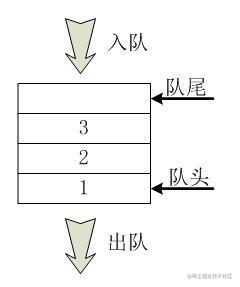Queue
``````public class Queue {
/***
* 1.单向队列（Queue）：只能在一端插入数据，另一端删除数据。
* 2.双向队列（Deque）：每一端都可以进行插入数据和删除数据操作。
*
*  与栈不同的是，队列中的数据不总是从数组的0下标开始的
*  选择的做法是移动队头和队尾的指针。
*  为了避免队列不满却不能插入新的数据，我们可以让队尾指针绕回到数组开始的位置，这也称为“循环队列”。
* */
// 单向循环队列，顺序存储结构实现
private Object[] objQueue;
//队列大小
private int maxSize;
//顶部
private int top;
//底部
private int bottom;
//实际元素
private int item;

public Queue(int size) {
maxSize = size;
objQueue = new Object[maxSize];
top = 0;
bottom = -1;
item = 0;
}

/**
* 入队
* @param obj
*/
if(item == maxSize){
throw new RuntimeException(obj+" add error, queue is full");
}
//循环队列，首尾结合，下标控制队首和队尾位置
if(bottom == maxSize-1){
bottom = -1;
}
objQueue[++bottom] = obj;
item++;
}

/**
* 出对
* @return
*/
public Object out(){
if(item == 0){
throw new RuntimeException("queue is null");
}
Object obj = objQueue[top];
//声明原顶栈可以回收空间(GC)
objQueue[top] = null;
top++;
//重置下标
if(top == maxSize){
top = 0;
}
item--;
return obj;
}

//链式存储结构实现
private class NodeQueue<Object>{
private Object data;

private NodeQueue next;

public NodeQueue(Object data, NodeQueue next) {
this.data = data;
this.next = next;
}
}
//队列头 出
private NodeQueue queueTop;
//队列尾 进
private NodeQueue queueBottom;
//队列大小
private int size;

public Queue() {
queueTop = null;
queueBottom = null;
size = 0;
}

/**
* 入队
* @param obj
*/
if(size == 0){
queueTop = new NodeQueue(obj,null);
//指向同一存储地址
queueBottom = queueTop;
}else{
NodeQueue<Object> nodeQueue = new NodeQueue(obj,null);
//让尾节点的next指向新增的节点
queueBottom.next = nodeQueue;
//以新节点作为尾节点
queueBottom = nodeQueue;
}
size ++;
}

/**
* 出队
* @return
*/
public Object removeNodeQueue(){
if(size == 0){
throw new RuntimeException("queue is null");
}
NodeQueue nodeQueue = queueTop;
queueTop = queueTop.next;
//声明原队列头next可以回收空间(GC)
nodeQueue.next = null;
size--;
return nodeQueue.data;
}

@Override
public String toString() {
StringBuilder sb = new StringBuilder("{ ");
for(NodeQueue nodeQueue = queueTop ; nodeQueue != null ; nodeQueue = nodeQueue.next){
sb.append(nodeQueue.data.toString()+" ");
}
return sb.toString()+"}";
}

public static void main(String[] args) {
Queue queue = new Queue();
System.out.println(queue);
queue.removeNodeQueue();
System.out.println(queue);
queue.removeNodeQueue();
queue.removeNodeQueue();
System.out.println(queue);
}
}
``````

• 链表即是由节点（Node）组成的线性集合，每个节点可以利用指针指向其他节点。它是一种包含了多个节点的、能够用于表示序列的数据结构。
• `单向链表`: 链表中的节点仅指向下一个节点，并且最后一个节点指向空。
• `双向链表`: 其中每个节点具有两个指针 p、n，使得 p 指向先前节点并且 n 指向下一个节点；最后一个节点的 n 指针指向 null。
• `循环链表`：每个节点指向下一个节点并且最后一个节点指向第一个节点的链表。
• 时间复杂度:
• 索引: `O(n)`
• 搜索: `O(n)`
• 插入: `O(1)`
• 移除: `O(1)`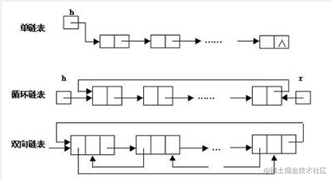Linked List
``````public class LinkedList {
/***
*/
private Node tail; //链表尾
private int size; //节点数

/**
* 双端链表
*/
public class Node{
private Object data;
private Node prev; //上一个
private Node next; //下一个

public Node(Object data) {
this.data = data;
}
}

this.tail = null;
this.size = 0;
}

/**
* 向链表头添加数据
* @param object
*/
Node node = new Node(object);
if(size == 0){
tail = node;
size++;
}else{
size++;
}
}

/**
* 删除头
*/
//头部指向下一个，prev值为null则说明是链表的头部
if(size != 0){
size--;
}
}

/**
*向链表尾添加数据
* @param object
*/
Node node = new Node(object);
if(size == 0){
tail = node;
size++;
}else{
node.prev = tail;
tail.next = node;
tail = node;
size++;
}
}

/**
* 删除尾部
*/
public void deleteTail(){
//尾部指向上一个，next值为null则说明是链表的尾部
if(size != 0){
tail.next = null;
tail = tail.prev;
size--;
}
}

/**
* 显示数据
*/
public void display(){
while (size > 0){
System.out.print("["+node.data+"->");
node = node.next;
size--;
}
}

public static void main(String[] args) {
}
}
``````

### Binary Tree

• `满二叉树`: 树中的每个节点仅包含 0 或 2 个节点。
• `完美二叉树（Perfect Binary Tree）`: 二叉树中的每个叶节点都拥有两个子节点，并且具有相同的高度。
• `完全二叉树`: 除最后一层外，每一层上的结点数均达到最大值；在最后一层上只缺少右边的若干结点。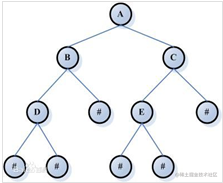Binary Tree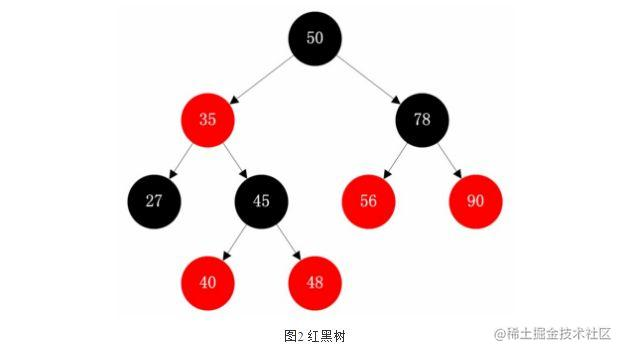红黑树(每节点五元素，颜色(如果一个节点是红的，则它的两个儿子都是黑的)、键值、左子节点、右字节的、父节点)

### Heap

• 堆(也被称为优先队列(队列+排序规则)，图一最大堆，图二最小堆)
• 堆是一种特殊的基于树的满足某些特性的数据结构，整个堆中的所有父子节点的键值都会满足相同的排序条件。堆更准确地可以分为最大堆与最小堆，在最大堆中，父节点的键值永远大于或者等于子节点的值，并且整个堆中的最大值存储于根节点；而最小堆中，父节点的键值永远小于或者等于其子节点的键值，并且整个堆中的最小值存储于根节点。
• 时间复杂度:
• 访问最大值 / 最小值: `O(1)`
• 插入: `O(log(n))`
• 移除最大值 / 最小值: `O(log(n))`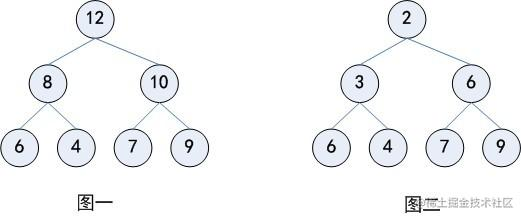### Hashing

• 哈希能够将任意长度的数据映射到固定长度的数据。哈希函数返回的即是哈希值，如果两个不同的键得到相同的哈希值，即将这种现象称为碰撞。
• Hash Map: Hash Map 是一种能够建立起键与值之间关系的数据结构，Hash Map 能够使用哈希函数将键转化为桶或者槽中的下标，从而优化对于目标值的搜索速度。
• 碰撞解决
• `链地址法（Separate Chaining）`: 链地址法中，每个桶是相互独立的，包含了一系列索引的列表。搜索操作的时间复杂度即是搜索桶的时间（固定时间）与遍历列表的时间之和。
• `开地址法（Open Addressing）`: 在开地址法中，当插入新值时，会判断该值对应的哈希桶是否存在，如果存在则根据某种算法依次选择下一个可能的位置，直到找到一个尚未被占用的地址。所谓开地址法也是指某个元素的位置并不永远由其哈希值决定。

### Graph

• 图是一种数据元素间为多对多关系的数据结构，加上一组基本操作构成的抽象数据类型。
• `无向图（Undirected Graph）`: 无向图具有对称的邻接矩阵，因此如果存在某条从节点 u 到节点 v 的边，反之从 v 到 u 的边也存在。
• `有向图（Directed Graph）`: 有向图的邻接矩阵是非对称的，即如果存在从 u 到 v 的边并不意味着一定存在从 v 到 u 的边。

### 快速排序

• 稳定: 否
• 时间复杂度:
• 最优时间: `O(nlog(n))`
• 最坏时间: `O(n^2)`
• 平均时间: `O(nlog(n))`

### 归并排序

• 归并排序是典型的分治算法，它不断地将某个数组分为两个部分，分别对左子数组与右子数组进行排序，然后将两个数组合并为新的有序数组。
• 稳定: 是
• 时间复杂度:
• 最优时间: `O(nlog(n))`
• 最坏时间: `O(nlog(n))`
• 平均时间: `O(nlog(n))`

### 桶排序

• 桶排序将数组分到有限数量的桶子里。每个桶子再个别排序（有可能再使用别的排序算法或是以递归方式继续使用桶排序进行排序）。
• 时间复杂度:
• 最优时间: `Ω(n + k)`
• 最坏时间: `O(n^2)`
• 平均时间:`Θ(n + k)`

### 基数排序

• 基数排序类似于桶排序，将数组分割到有限数目的桶中；不过其在分割之后并没有让每个桶单独地进行排序，而是直接进行了合并操作。
• 时间复杂度:
• 最优时间: `Ω(nk)`
• 最坏时间: `O(nk)`
• 平均时间: `Θ(nk)`

### 深度优先搜索

• 深度优先算法是一种优先遍历子节点而不是回溯的算法。
• 时间复杂度: `O(|V| + |E|)`

### 广度优先搜索

• 广度优先搜索是优先遍历邻居节点而不是子节点的图遍历算法。
• 时间复杂度: `O(|V| + |E|)`

### 拓扑排序

• 拓扑排序是对于有向图节点的线性排序，如果存在某条从 u 到 v 的边，则认为 u 的下标先于 v。
• 时间复杂度: `O(|V| + |E|)`

### Dijkstra 算法

• Dijkstra 算法 用于计算有向图中单源最短路径问题。
• 时间复杂度: `O(|V|^2)`

### Bellman-Ford 算法

• **`Bellman-Ford 算法`**是在带权图中计算从单一源点出发到其他节点的最短路径的算法。
• 尽管算法复杂度大于 Dijkstra 算法，但是它适用于包含了负值边的图。
• 时间复杂度:
• 最优时间: `O(|E|)`
• 最坏时间: `O(|V||E|)`

### Floyd-Warshall 算法

• `Floyd-Warshall 算法` 能够用于在无环带权图中寻找任意节点的最短路径。
• 时间复杂度:
• 最优时间: `O(|V|^3)`
• 最坏时间: `O(|V|^3)`
• 平均时间: `O(|V|^3)`

### Prim 算法

• **`Prim 算法`**是用于在带权无向图中计算最小生成树的贪婪算法。换言之，Prim 算法能够在图中抽取出连接所有节点的边的最小代价子集。
• 时间复杂度: `O(|V|^2)`

### Kruskal 算法

• **`Kruskal 算法`**同样是计算图的最小生成树的算法，与 Prim 的区别在于并不需要图是连通的。
• 时间复杂度: `O(|E|log|V|)`

### 位运算

• 位运算即是在位级别进行操作的技术，合适的位运算能够帮助我们得到更快地运算速度与更小的内存使用。
• 测试第 k 位: `s & (1 << k)`
• 设置第 k 位: `s |= (1 << k)`
• 第 k 位置零: `s &= ~(1 << k)`
• 切换第 k 位值: `s ^= ~(1 << k)`
• 乘以 2n: `s << n`
• 除以 2n: `s >> n`
• 交集: `s & t`
• 并集: `s | t`
• 减法: `s & ~t`
• 交换 `x = x ^ y ^ (y = x)`
• 取出最小非 0 位（Extract lowest set bit）: `s & (-s)`
• 取出最小 0 位（Extract lowest unset bit）: `~s & (s + 1)`
• 交换值: `x ^= y; y ^= x; x ^= y;`